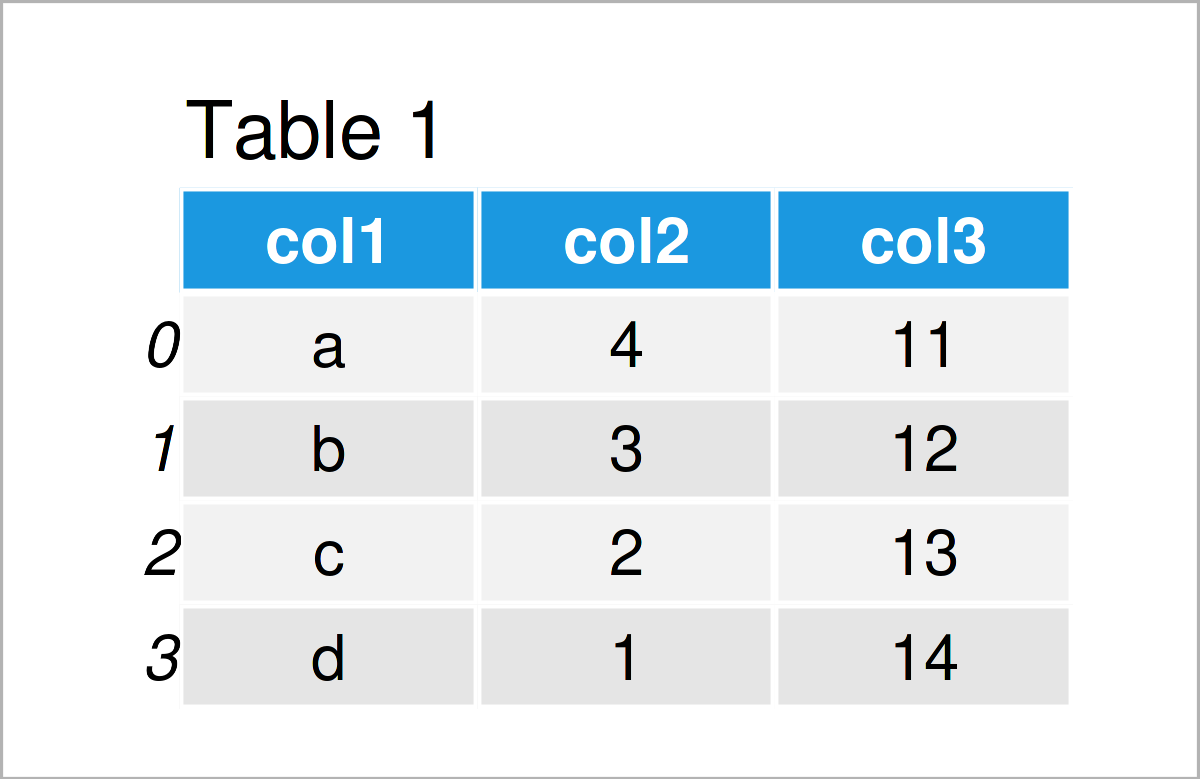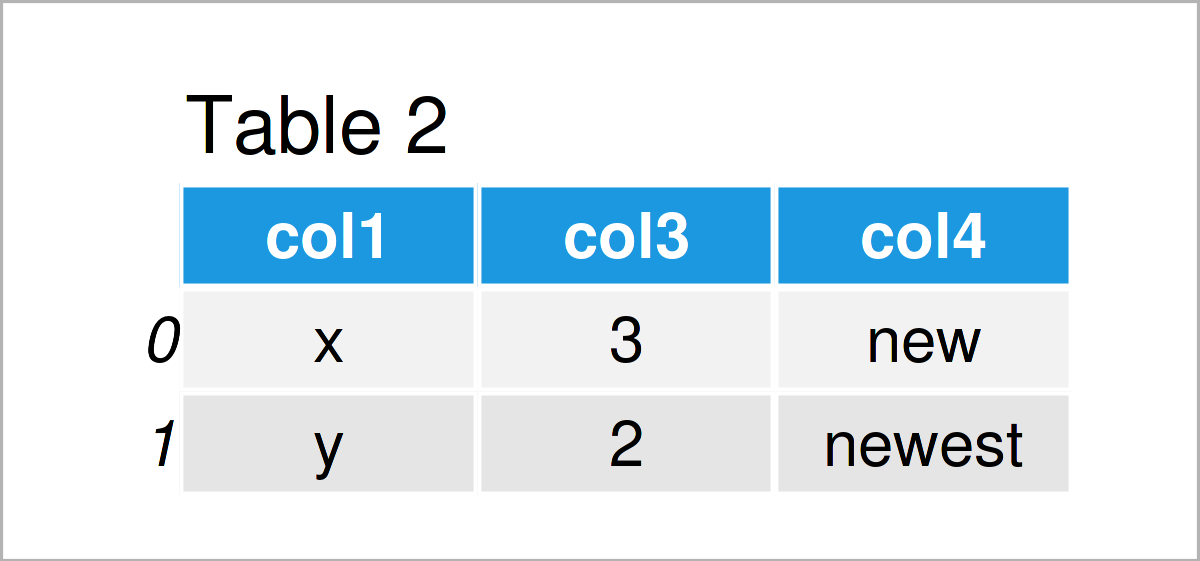# Combine pandas DataFrames with Different Column Names in Python (Example)

In this Python tutorial you’ll learn how to concatenate two pandas DataFrames with different columns.

The article will consist of this content:

Let’s dive right into the example…

## Example Data & Add-On Libraries

We first need to load the pandas library:

`import pandas as pd                                 # Import pandas library in Python`

Furthermore, consider the two example DataFrames below:

```data1 = pd.DataFrame({"col1":["a", "b", "c", "d"],  # Create first pandas DataFrame
"col2":range(4, 0, - 1),
"col3":range(11, 15)})
print(data1)                                        # Print first pandas DataFrame``````data2 = pd.DataFrame({"col1":["x", "y"],            # Create second pandas DataFrame
"col3":range(3, 1, - 1),
print(data2)                                        # Print second pandas DataFrame```Have a look at the two tables that got returned after running the previous syntax. It shows that our two example DataFrames are comprised of different columns.

The first data set called data1 contains the variables col1, col2, and col3; And the second data set called data2 consists of the columns with the names col1, col3, and col4.

Let’s merge these two DataFrames vertically!

## Example: Combine Two pandas DataFrames with Different Column Names Using concat() Function

The following syntax shows how to stack two pandas DataFrames with different column names in Python.

To achieve this, we can apply the concat function as shown in the Python syntax below:

```data_concat = pd.concat([data1, data2],             # Append two pandas DataFrames
ignore_index = True,
sort = False)
print(data_concat)                                  # Print combined DataFrame```As shown in Table 3, we have created a new pandas DataFrame that contains the values of our two input DataFrames.

Note that the concat function has inserted NaN values in case a column didn’t exist in both input DataFrames.

Furthermore, please note that we could apply the same syntax even to more than two DataFrames. We could also combine and append multiple DataFrames, no matter if it’s three, four, or five data sets!

## Video & Further Resources

Do you need further information on the Python syntax of this page? Then I recommend watching the following video tutorial on my YouTube channel. In the video, I demonstrate the contents of this article:

Please accept YouTube cookies to play this video. By accepting you will be accessing content from YouTube, a service provided by an external third party.If you accept this notice, your choice will be saved and the page will refresh.

Furthermore, you could have a look at the related tutorials on my website:

In summary: In this tutorial, I have illustrated how to combine two pandas DataFrames with different column names in the Python programming language. In case you have additional questions or comments, please let me know in the comments.

Subscribe to the Statistics Globe Newsletter